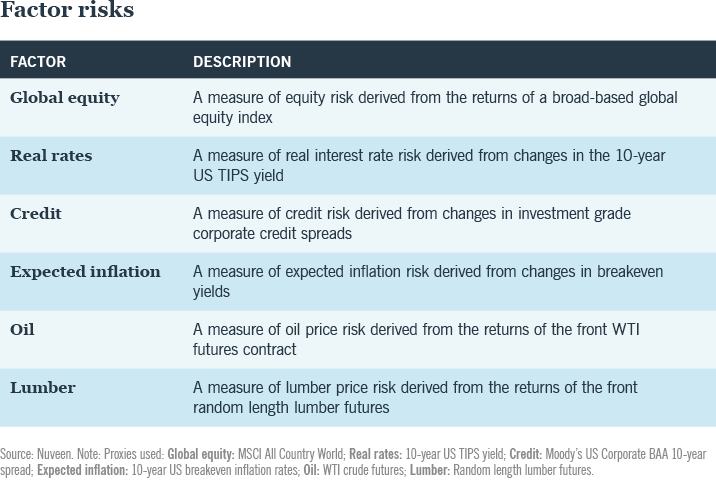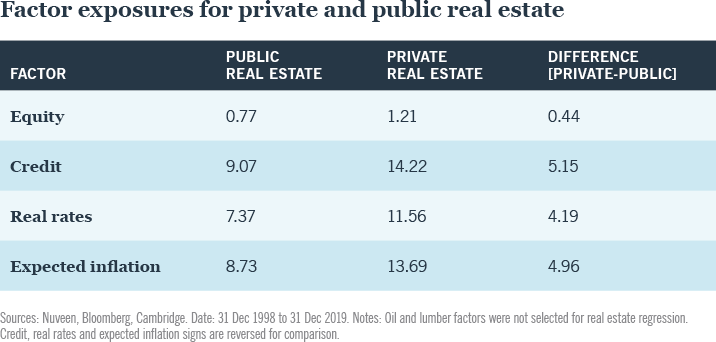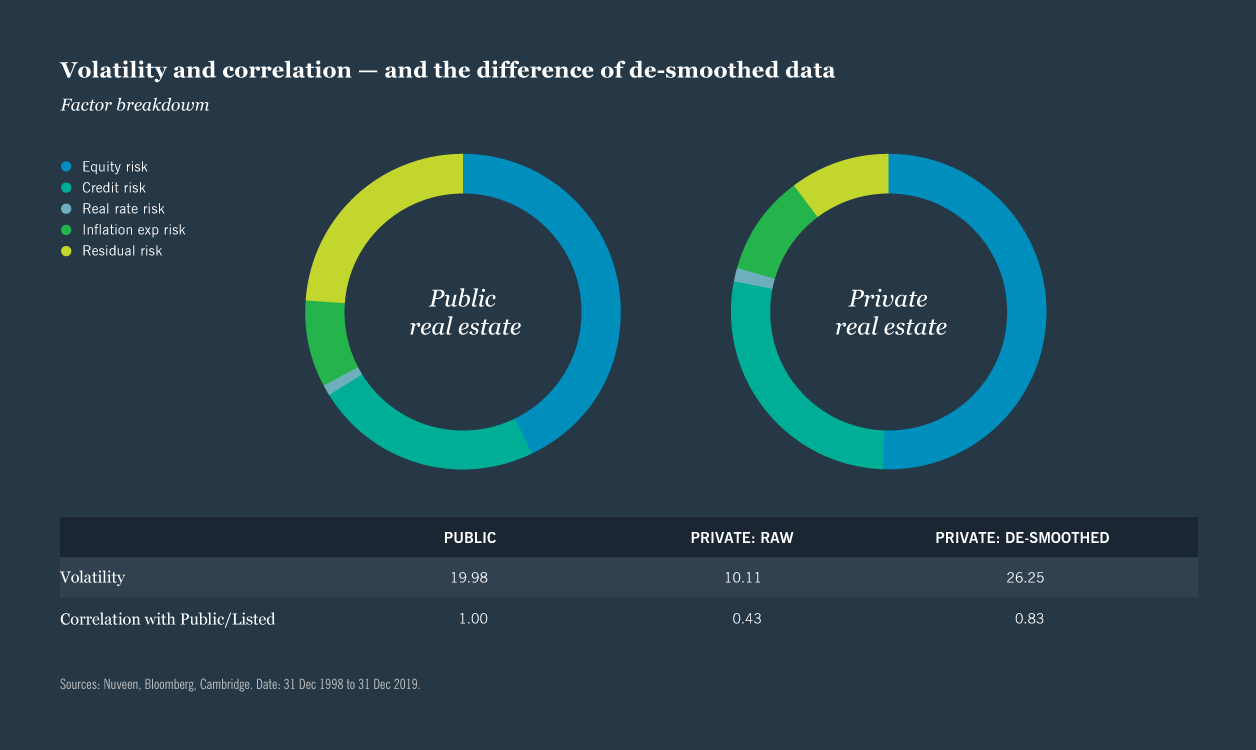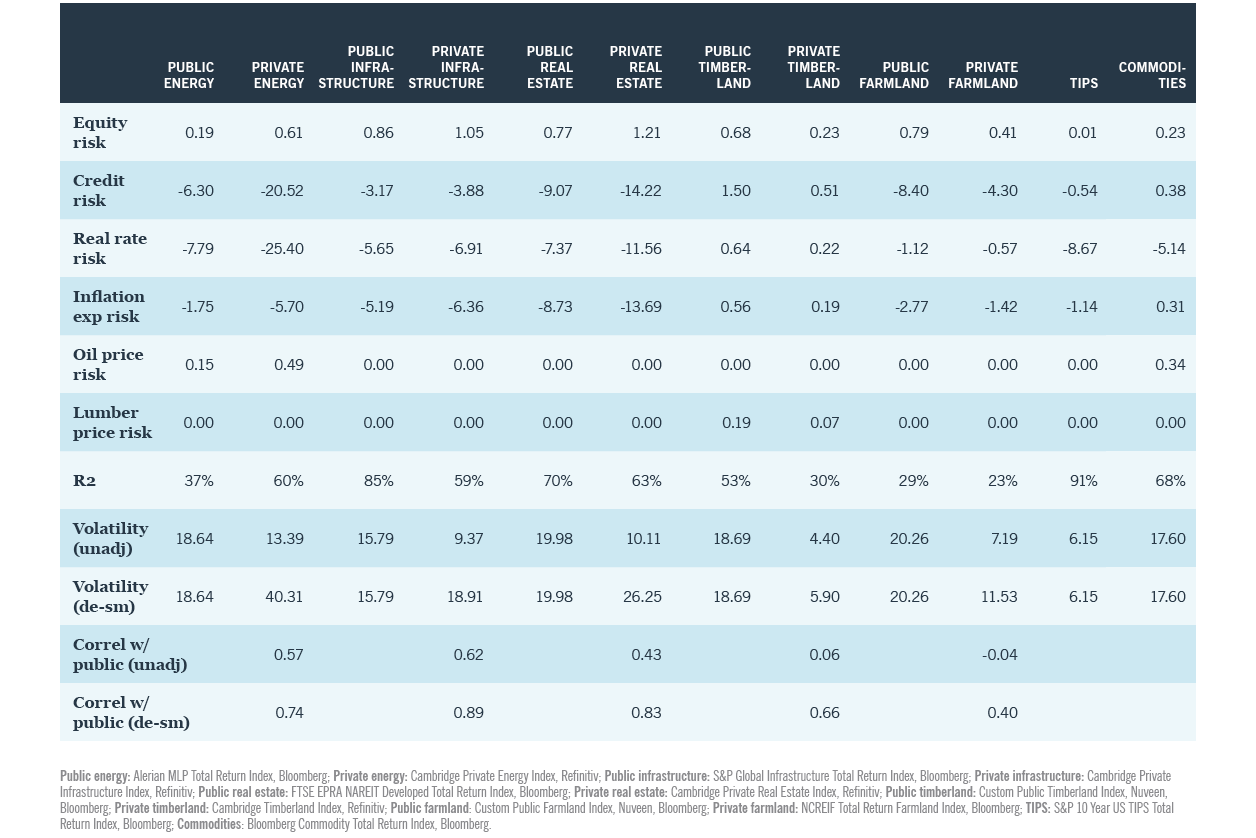Which type of investor are you?
Real assets

# The factors driving real asset returns## Identifying the factors driving real assets

Our factor analysis assumes investors are aiming to maximize the diversification benefits of real assets and also the return premiums specific to those assets.

We used common macro factors that drive a substantial proportion of risk in institutional portfolios — namely global equity, real rates, credit, expected inflation and oil. We also considered lumber as a factor exposure that is likely to feature in a timberland portfolio. The figure below provides a definition of each factor.

The exercise revealed the degree of common factor exposures across a range of public and private real assets, using indexes as proxies for both public and private real asset returns. The following section uses real estate to illustrate the factor exposure analysis that was performed for all private assets in this paper. To allow for proper comparison, this factor-based approach de-smoothed the private returns.### The common factors driving real estate

We deployed a three-step process to estimate the common factor exposures for a private real asset strategy:
1. Regress public index returns onto selected common factors
2. Regress reported private return series onto lagged (up to three years) common series returns
3. Derive factor exposure estimates from lagged common factor exposures
We used the FTSE EPRA NAREIT Developed index as the public index and the Cambridge Real Estate Index as a proxy for private real estate. We found that common factors explain 63% of the variation in returns and collectively are 79% correlated with the quarterly private real estate returns.

Based on the series of regressions, we estimated the exposures for private and public real estate displayed in the below figure.Using the results of our factor analysis, we de-smoothed private real estate returns and compared the risk factors driving public and private real estate.

###### One of the benefits of this analysis is it can be adapted to individual investor requirements.

As the below figure shows, the resulting volatility and correlation figures were understated using the raw data. After de-smoothing the returns of private real estate, we found the volatility of the assets increased more than two and a half times, from 10% to 26%. Its correlation with REITs almost doubled, from 0.43 to 0.83. These results are significant given how volatility and correlation data drive asset allocation decisions. Using the raw, unadjusted data could result in suboptimal allocations to real assets and understated risk forecastsOne of the benefits of this analysis is it can be adapted to individual investor requirements. As mentioned, in this exercise, we used the Cambridge Real Estate Index, which is composed of value-add and opportunistic real estate strategies. Investors who allocate solely to core private real estate strategies can use a different index that reflects their investment preferences (e.g., NCREIF ODCE).

### Findings: More than just common macro factors

•  In the analysis of public real estate, 70% of the return variation was explained by common macro factors of equity, credit, real rate and expected inflation. The remaining 30% indicated the existence of a real estate factor and other factors not captured by the common factor set.
• Analyzing private real estate in a similar way, we found a material amount of variation (63%) was explained by the same factors that drive public real estate and that there was evidence of an illiquidity and a pure real estate factor.

### Factor analysis across public and private real assets

We conducted similar analyses across a range of public and private real assets with the results shown in the figure below. Interesting to note is the difference in volatility and correlation figures for private real assets when using unadjusted data compared with de-smoothed data. In every case, they increase.### Uncommon factors — analysis of the residuals

Having determined how much of the common factors explain public and private real asset returns, the next stage in our analysis explored the residual (i.e., the variation in returns not explained by the common factors). As before we use real estate in the example.

Our hypothesis is that the residual is a function of three things:

• A pure real estate return component that is specific to the real asset and unrelated to the common factors
• An illiquidity (or private) return component, which represents the premium or discount from taking illiquidity risk
• Other unexplained factors
This breakdown not only helps investors understand the risks that are specific to the sub asset class, but whether there is a premium associated with those risks. In other words, does the investor believe they will be adequately compensated for taking those risks?

To help answer this, we analyzed the relationship between capitalization rates and private and public real estate performance (see appendix in research paper for details). We found evidence that the residual can be sufficiently explained by an asset-specific real estate factor common to both private and public real estate indices, and also a private, or illiquidity, factor that helps explain performance differences between public and private real estate.

These relationships can help investors estimate the component premiums to determine the attractiveness of the sub asset class and whether illiquidity risk is likely to be sufficiently compensated.

They provide strong evidence that real assets can play a beneficial role in investor portfolios. They offer a source of risk distinct from other assets in the portfolio, aiding diversification, and they offer compensation for taking on that risk.

## Continue reading: Think real assets

Real assets

Real assets

Real assets

#### Optimizing pension plan outcomes